Next: Minute No. 4, the Up: Quantum Field Theory in Previous: Minute No. 2, the

Minute No. 3, the Classical Field Theory

The classical field theory Lan70 describes certain physical systems as infinite-dimensional classical objects whose states need as many classical coordinates as there are points in the 3D space. Therefore, index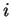that two minutes ago was used to enumerate the classical coordinates, now changes into the space point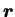, and the coordinate itself - into the value of a certain function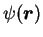, called the field, at point,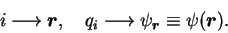(10)

Local Lagrangian density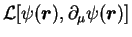defines the Lagrangian,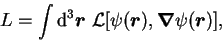(11)

and the extremal-action principle (2) gives the same Euler-Lagrange equations, which are now called field equations. Since the Lagrangian now depends on spatial derivatives of fields, the field equations are differential equations both in time and space. They can be, in principle, solved once the initial fields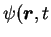=0) and spatial boundary conditions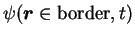are known. One thus obtains the complete past and future history of the system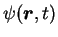. The rest is just a technicality ;) of how to solve differential equations.

In the physical world, the classical fields described above replace forces that act between particles. The whole Universe is thus composed of (classical) particles and (classical) fields. Particles are sources of fields, and fields exert forces on particles. The novelty here is the notion that a particle does not feel'' other particles; it only feels the fields generated by other particles. The so-called action at a distance disappeared from the theory, and a change of position of one particle, influences other particles only after the field it generates propagates to the rest of the world.

It is clear that the classical field theory is tailored to address the question of time propagation of fields, and makes the full sense within the relativistic approach where all fields propagate with one common and unchangeable velocity. Classical electrodynamics and classical gravity are theories of this type. Relativistic invariance takes then the place of a basic ingredient of the theory, and, e.g., action corresponding to Lagrangian (11) is manifestly relativistically invariant,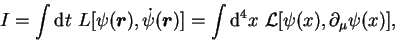(12)

because the four-dimensional volume element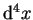is relativistically invariant. Here we introduced the standard four-vector notation of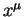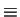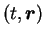and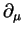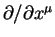.Next: Minute No. 4, the Up: Quantum Field Theory in Previous: Minute No. 2, the
Jacek Dobaczewski 2003-01-27